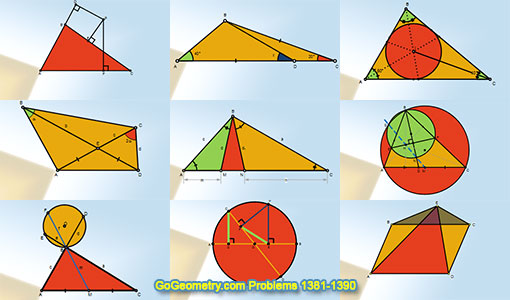# Geometry Problems 1381-1390. Level: High School, CollegeGeometry Problems 1381 - 1390Geometry Problem 1381.Parallelogram, Exterior Point, Triangle, Area. Geometry Problem 1382.Circle, Diameter, Radius, Perpendicular, Congruence. Geometry Problem 1383.Triangle, Median, Circle, Chord, Secant, Sum, Product. Geometry Problem 1384.Triangle, Orthocenter, Circle, Circumcircle, Angle Bisector, 90 Degree. Geometry Problem 1385.Triangle, Orthocenter, Circle, Circumcircle, Angle Bisector, Midpoint, Collinear Points. Geometry Problem 1386.Thabit ibn Qurra (826 -901) Theorem and more conclusions, generalized Pythagorean Theorem to any triangle. Geometry Problem 1387.Quadrilateral, Double Angle, Congruence, Isosceles Triangle. Geometry Problem 1388.Triangle 40-60-80 degree, Incenter, Congruence. Geometry Problem 1389.Triangle, 40-120-20 degree, Angle, Congruence. Geometry Problem 1390.Triangle, any point, perpendicular, sum of the squares.

Home | Sitemap | Geometry | Problems | Visual Index | 10 ProblemsEmail | Post a comment | by Antonio Gutierrez
Last updated: Jan 6, 2019